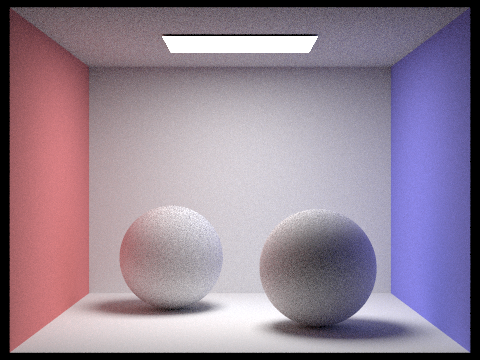In Part 3, we wrote our direct lighting function, but now we want to render images with full global illumination. Much of the visual richness in rendering comes from indirect lighting effects.

For Part 4, your job will be to implement the functionat_least_one_bounce_radiance and finish the function est_radiance_global_illumination in src/pathtracer/pathtracer.cpp.

• The function est_radiance_global_illumination is called to get an estimate of the total radiance with global illumination arriving at a point from a particular direction (e.g. on the image plane and going towards the image's center of projection). At the end of this part, this function will actually implement full global illumination.
• The function at_least_one_bounce_radiance is the main implementation work for this Part 4. At a high level, it should call the one_bounce_radiance function, and then recursively call itself to estimate the higher bounces. This recursive call should take one random sample of a direction based on the BSDF at the hit point, trace a ray in that sample direction, and recursively call itself on the new hit point.

### At a high level...

When we first enter this function, a ray left the camera and intersected the scene. We want to trace multi-bounce (inverse) paths, so we need to figure out where to go next.

Recall that this next location is actually where the light came from in order to arrive at the original intersection point. In direct lighting, we only cared if this next location was a light -- but now it could be anything!

If the next location is an object, then we need to estimate how much light arrived at that location -- and therein lies the recursion.Make sure you take a look at the pseudocode from the lecture slides on global illumination.

### Details: how do we stop infinite recursion?

Recall that our goal is to integrate over all paths of all lengths -- but this is computationally infeasible.

If energy dissipates, the contribution of higher bounces decreases exponentially.

• Russian Roulette provides us an unbiased method of random termination.
• In theory, the probability of terminating can be arbitrary -- but we suggest using a continuation probability between 0.6 or 0.7
• Remember that depending on the value you choose, your renders may look slightly different from ours or your peers -- but are the same in expectation.
• The max_ray_depth field tells you how many maximum bounces your ray should take. If it is > 1, then indirect illumination is "turned on" and we will always trace at least one indirect bounce (regardless of Russian Roulette).
• If the new ray doesn't intersect the scene, then it doesn't bounce off anything, and can't recurse.

### Code that will be useful

As in part 3, the starter code gives you:

• o2w and w2o, matrices for object-to-world space and world-to-object space transformations, respectively.
• These were created using the input isect's normal to calculate a local coordinate space for the object hit point. In this local space, the normal is $(0,0,1)$, so that $z$ is "up".
• Note that these are each the transpose and inverse of the other (they're orthonormal!)
• hit_p, the hit point of the ray, for convenience (note which coordinate space this is in).
• w_out, the outgoing direction in the local object frame.

Other functions that you will probably want to call:

• coin_flip(double p), from random_util.h
• This function returns true with probability p
• BSDF::sample_f(Vector3D& w_out, Vector3D* w_in, float* pdf)
• Recall from Part 3: This function requests the outgoing radiance direction w_out and returns the BSDF value as a Spectrum as well as 2 values by pointer. The values returned by pointer are
• the probabilistically sampled w_in unit vector giving the incoming radiance direction (note that unlike the direction returned by sample_L, this w_in vector is in the object coordinate frame!) and
• a pdf float giving the probability density function evaluated at the return w_in direction.
• BVHAccel::intersect(Ray&, Intersection*)
• at_least_one_bounce_radiance(Ray&, Intersection&) -- because of recursion!
• EPS_F, the epsilon offset

### Notes

• For the ray depth cutoff to work, you should initialize your camera rays' depths as max_ray_depth in raytrace_pixel, and modify this field with each recursive call.
• Remember that when generating rays originating from an existing hit point, offset the range of valid intersections with the value EPS_F.
• Don't forget all the multiplicative and normalization factors, as in Part 3
• Here, we additionally need to normalize by the continuation probability!

### Nice work!

You still can only render diffuse BSDFs until completing the first part of Project 3-2, however, you should now see some nice color bleeding in Lambertian scenes. You should be able to correctly render images such as

./pathtracer -t 8 -s 64 -l 16 -m 5 -r 480 360 -f spheres.png ../dae/sky/CBspheres_lambertian.dae(The last command takes 59 seconds for the reference solution on Hive)

Remember you will be running experiments for the write-up -- since the renders will be taking some time, it's a good idea to start rendering now!